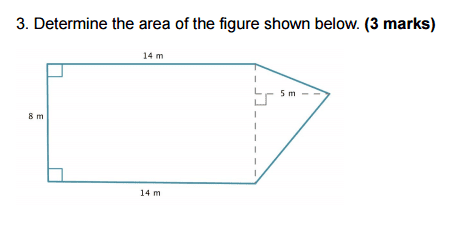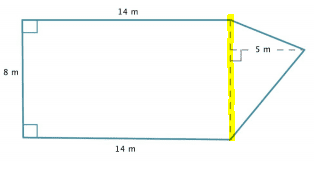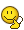# Area of Composite shapes.

• Kirito123
In summary, the conversation discusses finding the area of a composite shape using the formula A = L x W for a rectangle and A = hbb / 2 for a triangle. The person already knows the height and base of the triangle, but is unsure how to find the length of the remaining side. After receiving some clarification, they realize they can directly calculate the area of the triangle using the given measurements.

## Homework StatementA = l x w
A = hbb / 2

## The Attempt at a Solution

To find the area of a rectangle you would use the formula "A = L x W". In this case A = 14 x 8 which is eual to 112, Now all i need is the area of the triangle and then i add them together and that will be the area of the whole figure.

So i know we have the height of the triangle is 5
Also the part i colored yellow is 8 m long.So my question is how can i find the length of 1 of the sides of the triangle.

Kirito123 said:

## Homework Statement

View attachment 100649

A = l x w
A = hbb / 2

## The Attempt at a Solution

To find the area of a rectangle you would use the formula "A = L x W". In this case A = 14 x 8 which is eual to 112, Now all i need is the area of the triangle and then i add them together and that will be the area of the whole figure.

So i know we have the height of the triangle is 5
Also the part i colored yellow is 8 m long.
View attachment 100650

So my question is how can i find the length of 1 of the sides of the triangle.

Why would you need to find that?

Ray Vickson said:
Why would you need to find that?

I need to find the area of that triangle, that way i can find the whole area of that composite shape.

Kirito123 said:
I need to find the area of that triangle, that way i can find the whole area of that composite shape.
for area of that triangle you already have the base ( 8m) and height (5m), so you don't need to calculate other sides, you can directly calculate the area.

OMG I totally didn't even think about that...... face palm. sahil you just cleared my mind, man I am so in a blrr today. anyway i now know what to do.

Thanks m8 :)